# Samacheer Kalvi 6th Maths Solutions Term 1 Chapter 5 Statistics Ex 5.2## Tamilnadu Samacheer Kalvi 6th Maths Solutions Term 1 Chapter 5 Statistics Ex 5.2

Question 1.
Fill in the blanks.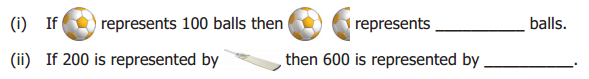(iii) Representation of data by using pictures is known as _______
Solution:
(i) 150 balls
(ii)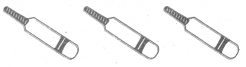(iii) Pictograph

Question 2.
Draw a pictograph for the give data.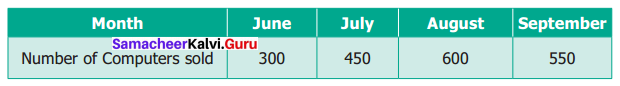Solution:
Pictograph of Number of computers sold.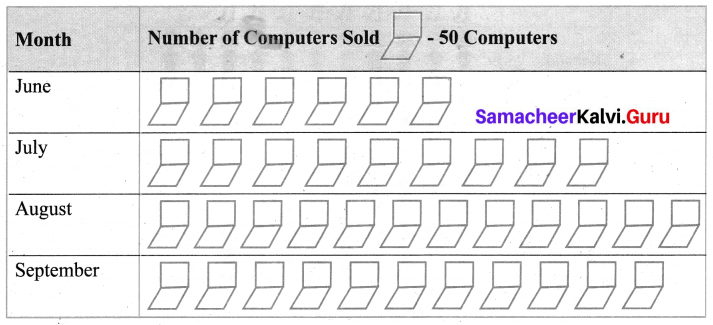Question 3.
The following table shows the number of tourists who visited the places in the month of May. Draw a pictograph.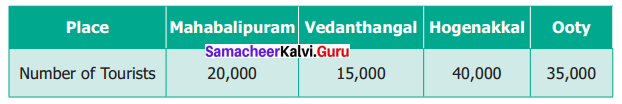Solution:
The pictograph for the number of tourists who visited various places: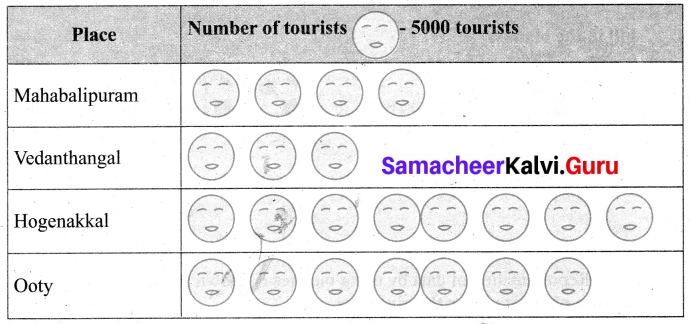Question 4.
The following pictograph shows the number of students playing different games in a school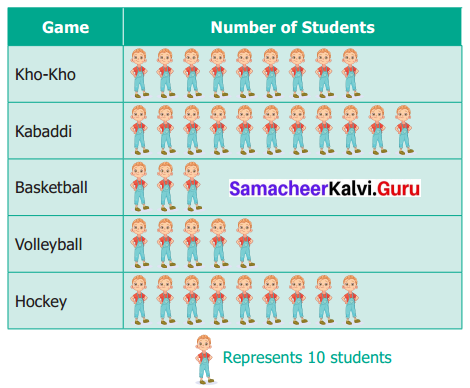(i) Which is the most popular game among the students?
(ii) Find the number of students playing kabaddi?
(iii) Which two games are played by an equal number of students?
(iv) What is the difference between the number of students playing Kho-Kho and Hockey?
(v) Which is the least popular game among the students?
Solution:
(i) Kabaddi is the most popular game among students.
(ii) There are 11 × 10 = 110 students playing kabaddi.
(iii) Kho-Kho and Hockey are played by an equal number of students.
(iv) Difference is 90 – 90 = 0.
(v) Basketball is the least popular game among students.Objective Type Questions

Question 5.
The representation of‘one picture to many objects’ in a pictograph is called
(a) Tally mark
(b) Pictoword
(c) Scaling
(d) Frequency
Solution:
(c) Scaling

Question 6.
The representation of ‘one picture too many objects’ in a Pictograph is called _____
(a) Tally mark
(b) Pictoword
(c) Scaling
(d) Frequency
Solution:
(c) Scaling

Question 7.
A Pictograph is also known as ______
(a) Pictoword
(b) Pictogram
(c) Pictophrase
(d) Pictograph
Solution:
(b) Pictogram